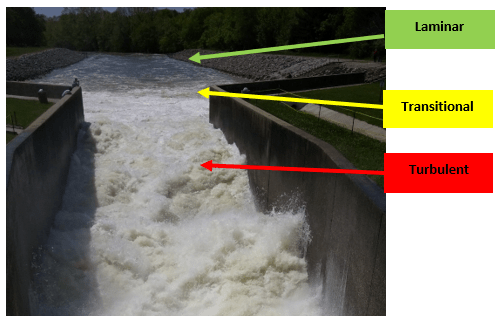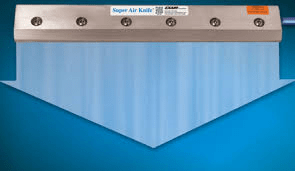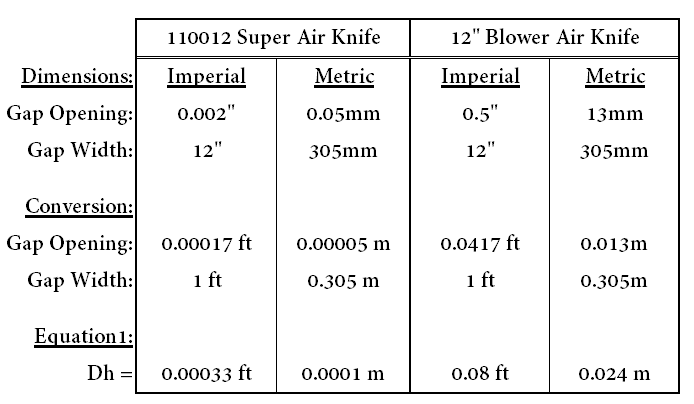## Video Blog: Laminar and Turbulent Flows

I have written blogs about laminar and turbulent flows as related to the Reynold’s number.  Now, let’s demonstrate the difference between the two flows and the advantages of laminar flow from EXAIR’s engineered air nozzles; as demonstrated by our VariBlast Safety Air Gun.

John Ball
Application Engineer
Email: johnball@exair.com
Twitter: @EXAIR_jb

## Laminar Flow Compared to Turbulent Flowturbulent vs laminar

Fluid mechanics is the field that studies the properties of fluids in various states.  There are two main areas; fluid statics and fluid dynamics.  Fluid dynamics studies the forces on a fluid, either as a liquid or a gas, during motion.  Osborne Reynolds, an Irish innovator, popularized this dynamic with a dimensionless number, Re. This number determines the state in which the fluid is moving; either laminar flow, transitional flow, or turbulent flow.  Equation 1 below shows the relationship between the inertial forces of the fluid as compared to the viscous forces.

Equation 1:  Re = V * Dh/u

Re – Reynolds Number (no dimensions)

V – Velocity (feet/sec or meters/sec)

Dh – hydraulic diameter (feet or meters)

u – Kinematic Viscosity (feet^2/sec or meter^2/sec)

The value of Re will mark the region in which the fluid (liquid or gas) is moving.  If the Reynolds number, Re, is below 2300, then it is considered to be laminar (streamline and predictable).  If Re is greater than 4000, then it is considered to be turbulent (chaotic and violent).  The area between these two numbers is the transitional area where you can have eddy currents and some non-linear velocities.  To better show the differences between each state, I have a picture below that shows water flowing from a drain pipe into a channel.  The water is loud and disorderly; traveling in different directions, even upstream.  With the high velocity of water coming out of the drain pipe, the inertial forces are greater than the viscous forces of the water.  This indicates turbulent flow with a Reynolds number larger than 4000.  As the water flows into the mouth of the river, the waves transform from a disorderly mess into a more uniform stream.  This is the transitional region.  A bit further downstream, the stream becomes calm and quiet, flowing in the same direction.  This is laminar flow.  Air is also a fluid, and it will behave in a similar way depending on the Reynolds number.Turbulent to Laminar Water

Why is this important to know?  In certain applications, one state may be better suited than the other.  For mixing, suspension and heat transfer; turbulent flows are better.  But, when it comes to effective blowing, lower pressure drops and reduced noise levels; laminar flows are better.  In many compressed air applications, the laminar region is the best method to generate a strong force efficiently and quietly.  EXAIR offers a large line of products, including the Super Air Knives, Super Air Amplifiers and Super Air Nozzles that utilizes that laminar flow for compressed air applications.  If you would like to discuss further how laminar flows could benefit your process, an EXAIR Application Engineer will be happy to help you.

John Ball
Application Engineer
Email: johnball@exair.com

Twitter: @EXAIR_jb

## What is Laminar Flow and Turbulent Flow?

Fluid mechanics is the field that studies the properties of fluids in various states.  There are two areas, fluid statics and fluid dynamics.  Fluid dynamics studies the forces in a fluid, either as a liquid or a gas, during motion.  Osborne Reynolds, an Irish innovator, popularized this dynamic with a dimensionless number, Reyonlds number. This number can indicate the different states that the fluid is moving; either in laminar flow or turbulent flow.  The equation below shows the relationship between the inertial forces of the fluid as compared to the viscous forces.  Reynolds number, Re, can be calculated by Equation 1:

Equation 1:  Re = V * Dh/u

Re – Reynolds Number (no dimensions)

V – Velocity (feet/sec or meters/sec)

Dh – hydraulic diameter (feet or meters)

u – Kinematic Viscosity (feet^2/sec or meter^2/sec)

The value of Re will mark the region in which the fluid (liquid or gas) is moving.  If the Reynolds number, Re, is below 2300, then it is considered to be laminar (streamline and predictable).  If Re is greater than 4000, then the fluid is considered to be turbulent (chaotic and violent).  The area between these two numbers is called the transitional area where you can have small eddy currents and some non-linear velocities.  To better show the differences between each state, I have a picture below that shows water flowing from a drain pipe into a channel.  The water in the channel is loud and disorderly; traveling in different directions, even upstream.  With the high speed coming from the drain pipe, the inertial forces are greater than the viscous forces of the water.  The Reynolds number is larger than 4000 which indicates turbulent flow.  As the water travels into the mouth of the river after the channel, the waves transform from a disorderly mess into a more uniform stream.  This is the transitional region.  A bit further downstream, the stream becomes calm and quiet, flowing in the same direction.  This is the laminar flow region where Re is less than 2300.  Air, like the water in the picture, is also a fluid, and it will behave exactly in the same way depending on the Reynolds number.Turbulent to Laminar Flows

Why is this important to know?  In certain applications, one state may be better suited than the other.  For mixing, particle suspension and heat transfer; turbulent flows are needed.  But, when it comes to effective blowing, lower pressure drops and lower noise levels; laminar flows are required.  In many compressed air applications, the laminar flow region is the best area to use compressed air.  EXAIR offers a large line of products, including the Super Air Knives and Super Air Nozzles that uses that laminar flow to generate a strong force efficiently and quietly.  If you would like to discuss further how laminar flows could benefit your process, an EXAIR Application Engineer will be happy to assist you.

John Ball
Application Engineer
Email: johnball@exair.com
Twitter: @EXAIR_jb

## Laminar Flow vs. Turbulent Flow – Calculations and ExamplesSuper Air Knife

What is laminar flow and turbulent flow?  Osborne Reynolds popularized this phenomenon with a dimensionless number, Re. This number is the ratio of the inertial forces to the viscous forces.  If the inertial forces are dominant over the viscous forces, the fluid will act in a violent and chaotic manner.  The formula to determine the Reynolds number is as follows:

Equation 1:  Re = V * Dh/u

Re – Reynolds Number (no dimensions)

V – Velocity (Feet/sec or Meters/sec)

Dh – hydraulic diameter (Feet or Meters)

u – Kinematic Viscosity (Feet^2/sec or Meter^2/sec)

The value of Re will determine the state in which the fluid (liquid or gas) will move.  If the Reynolds number, Re, is below 2300, then it is considered laminar (streamline and predictable).  If Re is greater than 4000, then it is considered turbulent (chaotic and disarrayed).  The area between these two numbers is the transitional area where you start to get small eddy currents and velocities in a non-linear direction.  When it comes to effective blowing, cleaning and lower noise levels, laminar flow is optimal.

Let’s do a comparison of Reynolds numbers between the EXAIR Super Air Knife and a blower-type air knife.  Both products are designed to clean and blow off wide areas like conveyor belts.  The EXAIR Super Air Knife is powered by compressed air, and the blower-type air knife is powered by an air blower.  The main difference between the two products is the dimension of the slot opening.  The Super Air Knife has a gap opening of 0.002″ (0.05mm).  It uses the force of the compressed air to “push” it through the small opening to create a strong velocity.  A blower does not generate a high force, so the opening of the blower-type air knife has to be larger to overcome any back pressure the opening creates.  The gap opening is typically 0.5″ (13mm).  From Equation 1 above, the gap opening helps determine the hydraulic diameter, Dh.  The hydraulic diameter is an equivalent tube diameter from a non-circular flow area.  Since both the Super Air Knives and blower-type air knives have rectangular cross sections, the Dh can be calculated as follows:

Equation 2: Dh = 2 * a * b/ (a + b)

Dh – Hydraulic Diameter (feet or meter)

a – Gap Opening (feet or meter)

b – Gap Width (feet or meter)

If we compare for example a standard 12″ wide air knife, we can calculate the hydraulic diameter, Dh, by using Equation 2:Hydraulic Diameter Calculations

The exit velocity of the Super Air Knives can be changed by regulating the air pressure.  The higher the air pressure, the higher the velocity.  The blower type air knives can use a blower with a variable frequency drive (VFD) to change the exit velocity .  A reasonable air pressure for the Super Air Knife is 80 PSIG, and the exit velocity is near 540 ft/sec (164 m/s).  To equate this to a blower system, the size of the blower will determine the maximum velocity.  To do this comparison, I will use the same velocity as the Super Air Knife.  With the kinematic viscosity of air, it has a value of 0.000164 ft^2/sec (0.000015 m^2/sec) at 70 deg. F (21 deg C).  Now we have all the information for the comparison, and we can now find the Reynolds number from Equation 1:Reynolds Number Calculation

As you can see from the above calculations, the Super Air Knife has a Reynolds number, Re, below 2300.  The flow characteristic is in the region of laminar (predictable and streamline).  The blower air knife has a Reynolds number, Re, above 4000.  The flow dynamic coming out of the blower-type air knife is turbulent (chaotic and disoriented).  To better show the difference in laminar flow and turbulent flow, I have a picture below that shows both states with water as a fluid (being that air is an invisible fluid).   Here is an example of water  coming out of a drain pipe at Cave Run Lake (first picture below).  With the inertial forces much higher than the viscosity of the water, it is in a turbulent state;  loud and disorderly.  Reynolds number is greater than 4000.  The water is traveling in different directions, even upstream.  As the water flows into the mouth of the river after the channel (second picture below), the waves transform from a violent mess into a quiet, calm stream flowing in the same direction.  This is laminar flow (Re is less than 2300).Turbulent Water from PipeFrom Channel to River

With the engineered design of the Super Air Knife, the thin slot helps to create that laminar flow.  All the air is moving in the same direction, working together to give a higher force to remove debris.  If you have turbulent flow like that of a blower air knife, the noise level is much higher, and the disoriented forces are less effective in blowing.  Turbulence is useful for mixing, but horrible for trying to clean or wipe a conveyor belt.  If you have any open pipes, drilled pipes or blower-type air knives in your application, you should try an EXAIR product to see the difference.  An Application Engineers can help you take advantage of laminar airflow.

John Ball
Application Engineer
Email: johnball@exair.com
Twitter: @EXAIR_jb